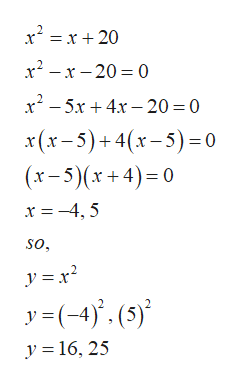# Solve the following system of equations by the substitution method. y=x2y=x+20what is the solution set

Question
14 views

Solve the following system of equations by the substitution method.

y=x2

y=x+20

what is the solution set

check_circle

Step 1

Given information

Step 2

To determine solution sets by substitution method

Step 3

Calculation

Put value of y from equa...help_outlineImage Transcriptionclosexx20 x2-x20 0 x2-5x4x-20 0 (x-5)+4(x-5)0 (x-5)(x+4) 0 x =-45 so y(-4).(5) y 16, 25 fullscreen

### Want to see the full answer?

See Solution

#### Want to see this answer and more?

Solutions are written by subject experts who are available 24/7. Questions are typically answered within 1 hour.*

See Solution
*Response times may vary by subject and question.
Tagged in

### Algebra